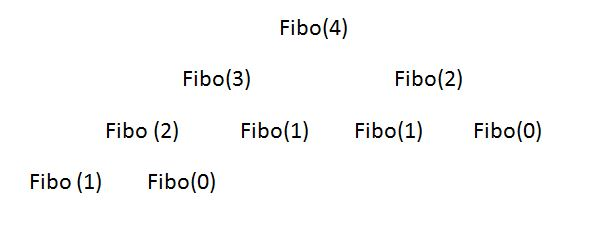Q:

# C++ program to find Fibonacci number using different methods

Fibonacci numbers are the numbers having a specific sequential pattern.

0 , 1 , 1 , 2 , 3 , 5 , 8 , 13 , 21 , 34, ... this type of sequence follow a mathematical pattern.

Fn = Fn-1 + Fn-2 , where F0 = 0 , F1 = 1

Where n = 2,3,4,5,6,7,8, ...

### 1. Using recursion method

In recursion a lot work is done as it repeatedly calculates the number and solves same case more than one time.

For Example:As it calculates Fibo(1) & Fibo(0) multiple time

Consider the program:

``````#include<iostream>
using namespace std;

int Fib(int n)
{
if(n<=1)
return n;
return Fib(n-1)+Fib(n-2);	/*recusively add the numbers*/
}

int main()
{
int n;  /*position of the element starting from 0...n*/
cout<<"Enter the number :";
cin>>n;
cout<<"Number at "<<n<<"th place is "<<Fib(n)<<endl;
return 0;
}``````

Output

```Enter the number :10
Number at 10th place is 55
```

### 2. Using Dynamic programming method

A dynamic programming algorithm remembers the past result and uses them to find new result means it solve complex problems by breaking it down into a collection of simpler subproblems, then solving each of those subproblems only once ,and storing their solution for future use instead of recomputing their solutions again.

Algorithm:

``````Fib(n)
if n=0
return 0
else
prev_Fib=0,curr_Fib=1
repeat n-1 times  /*if n=0 it will skip*/
next_Fib=prev_Fib+curr_Fib
prev_Fib=curr_Fib
curr_Fib=next_Fib
return curr_Fib``````

Consider the program:

``````#include<iostream>
using namespace std;

int main()
{
int n;
cout<<"Enter the number :";
cin>>n; /*position of the element starting from 0...n*/

int Fibo[n+1],i;
Fibo=0,Fibo=1;

for(i=2;i<=n;i++)
{
Fibo[i]=Fibo[i-1]+Fibo[i-2];  /*It will store the sum of previous two elements */
}
cout<<"Number at "<<n<<"th place is "<<Fibo[n]<<endl;

return 0;
}``````

Output

```Enter the number :10
Number at 10th place is 55```

### 3. Without using array

Well it is the easiest way to calculate Fibonacci number as we just have to add two previous numbers to calculate next number.

Consider the program:

``````#include<iostream>
using namespace std;

int Fib(int n)
{
if(n==0)
return 0;
else
{
int prev_Fib=0,curr_Fib=1,next_Fib;
if(n==0)
return n;
while(n>=2)
{
next_Fib=prev_Fib+curr_Fib;
prev_Fib=curr_Fib;
curr_Fib=next_Fib;
n--;
}
return curr_Fib;
}
}

int main()
{
int n;   /*position of the element starting from 0...n*/
cout<<"Enter the number :";
cin>>n;
cout<<"Number at "<<n<<"th place is "<<Fib(n)<<endl;
return 0;
}``````

Output

```Enter the number :10
Number at 10th place is 55```